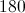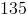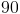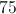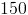## Example Questions

← Previous 1 3

### Example Question #1 : Other Polygons

If the following shape was going to be drawn in a circle, what is the minimum radius of the circle?

8

11

7

9

10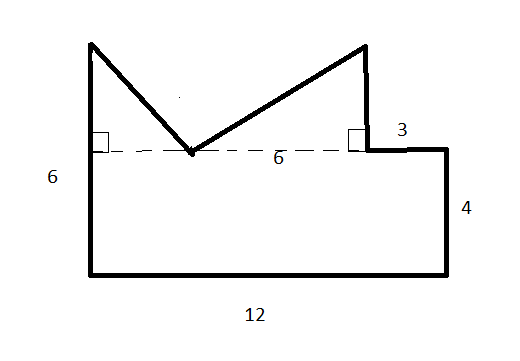7

Explanation:

IF you draw the longest diagonal across the shape, the length of it is 13.4. This means the radius must be at least 6.7. The answer is 7.

### Example Question #2 : Other Polygons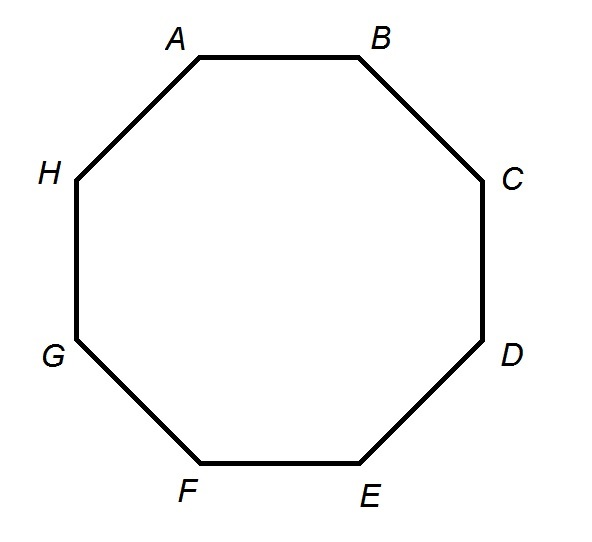Each side of the above octagonal track is 264 feet in length. Julie starts at point A and runs clockwise at a steady speed of nine miles an hour for nine minutes. When she is finished, which of the following points is closest to her?

Point D

Point E

Point F

Point G

Point C

Point D

Explanation:

Julie runs for nine minutes, or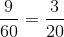hour; she runs nine miles per hour. Setting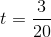and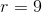in the rate formula, we can evaluate distance in miles: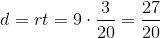Julie runs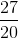miles, which converts to feet by multiplication by 5,280 feet per mile: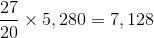feet.

Each side of the octagonal track measures 264 feet, so Julie runs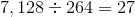sides of the track; this is equivalent to running the entire track three times, then three more sides. She is running clockwise, so three more sides from Point A puts her at Point D. This is the correct response.

### Example Question #3 : Other Polygons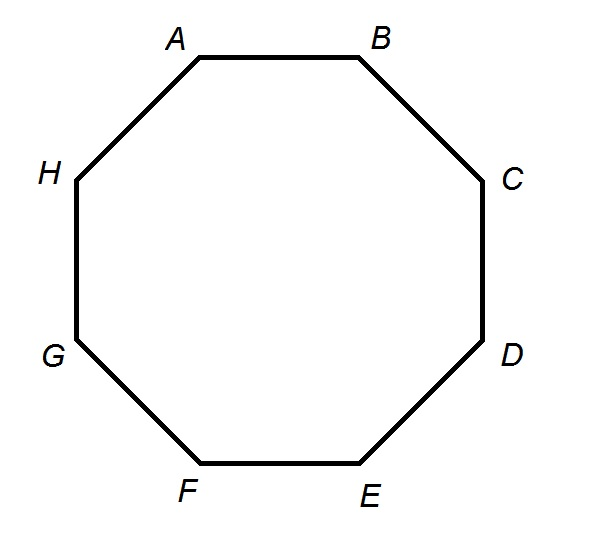Plato High School has an unusual track in that it is shaped like a regular octagon. The track has a perimeter of two-fifths of a mile.

Boris starts at Point A and runs clockwise until he gets halfway between Point E and Point F. Which of the following responses comes closest to the number of feet he runs?

1,100 feet

1,400 feet

1,200 feet

1,500 feet

1,300 feet

1,200 feet

Explanation:

One mile comprises 5,280 feet; the perimeter of the track, two-fifths of a mile, is equal to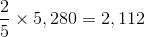feet.

Each (congruent) side of the octagonal track measures one-eighth of this,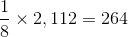feet.

By running clockwise from Point A to halfway between Point E and Point F, Boris runs along four and one half sides, each of which has this length, for a total running distance of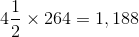feet.

Of the five responses, 1,200 comes closest.

### Example Question #1 : How To Find An Angle In A Polygon

A seven sided polygon has a side length of 14”.  What is the measurement of one of the interior angles of the polygon?

252 degrees

154.28 degrees

257.14 degrees

180 degrees

128.57 degrees

128.57 degrees

Explanation:

The formula for of interior angles based on a polygon with a number of side n is:

Each Interior  Angle = (n-2)*180/n

= (7-2)*180/7 = 128.57 degrees

### Example Question #1 : How To Find An Angle In A Polygon

If angle A and angle C are complementary angles and B and D are supplementary angles, which of the following must be true?

A * C > B * D

A/D < B/C

Explanation:

This question is very misleading, because while each answer COULD be true, none of them MUST be true. Between angle A and C, onne of the angles could be very small (0.001 degrees) and the other one could be very large. For instance, if A = 89.9999 and C = 0.0001, AC = 0.009. On the other hand, the two angles could be very siimilar. If B = 90 and D = 90 then BD = 8100 and BD > AC. If we use these same values we disprove AD = BC as 8100 ≠ .009. Finally, if B is a very small value, then B/C will be very small and smaller than A/D.

### Example Question #2 : How To Find An Angle In A Polygon

In isosceles triangle ABC, the measure of angle A is 50 degrees.  Which is NOT a possible measure for angle B?

50 degrees

There is more than one correct answer

80 degrees

65 degrees

95  degrees

95  degrees

Explanation:

If angle A is one of the base angles, then the other base angle must measure 50 degrees. Since 50 + 50 + x = 180 means x = 80, the vertex angle must measure 80 degrees.

If angle A is the vertex angle, the two base angles must be equal. Since 50 + x + x = 180 means x = 65, the two base angles must measure 65 degrees.

The only number given that is not possible is 95 degrees.

### Example Question #1 : How To Find An Angle In A Polygon

In triangle ABC, the measure of angle A = 70 degrees, the measure of angle Bx degrees, and the measure of angle Cy degrees. What is the value of y in terms of x?

70 – x

110 + x

x – 70

70 + x

110 – x

110 – x

Explanation:

Since the three angles of a triangle sum to 180, we know that 70 + x + y = 180. Subtract 70 from both sides and see that x + y = 110. Subtract x from both sides and see that y = 110 – x.

### Example Question #1 : How To Find An Angle In A Polygon

What is the measure, in degrees, of each interior angle of a regular convex polygon that has twelve sides?

135

150

120

180

175

150

Explanation:

The sum of the interior angles, in degrees, of a regular polygon is given by the formula 180(n – 2), where n is the number of sides. The problem concerns a polygon with twelve sides, so we will let n = 12. The sum of the interior angles in this polygon would be 180(12 – 2) = 180(10) = 1800.

Because the polygon is regular (meaning its sides are all congruent), all of the angles have the same measure. Thus, if we divide the sum of the measures of the angles by the number of sides, we will have the measure of each interior angle. In short, we need to divide 1800 by 12, which gives us 150.

### Example Question #1 : How To Find An Angle In A Polygon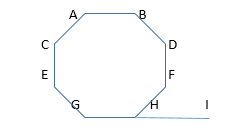In the figure above, polygon ABDFHGEC is a regular octagon. What is the measure, in degrees, of angle FHI?

40

30

50

45

60

45

Explanation:

Angle FHI is the supplement of angle FHG, which is an interior angle in the octagon. When two angles are supplementary, their sum is equal to 180 degrees. If we can find the measure of each interior angle in the octagon, then we can find the supplement of angle FHG, which will give us the measure of angle FHI.

The sum of the interior angles in a regular polygon is given by the formula 180(n – 2), where n is the number of sides in the polygon. An octagon has eight sides, so the sum of the angles of the octagon is 180(8 – 2) = 180(6) = 1080 degrees. Because the octagon is regular, all of its sides and angles are congruent. Thus, the measure of each angle is equal to the sum of its angles divided by 8. Therefore, each angle in the polygon has a measure of 1080/8 = 135 degrees. This means that angle FHG has a measure of 135 degrees.

Now that we know the measure of angle FHG, we can find the measure of FHI. The sum of the measures of FHG and FHI must be 180 degrees, because the two angles form a line and are supplementary. We can write the following equation:

Measure of FHG + measure of FHI = 180

135 + measure of FHI = 180

Subtract 135 from both sides.

Measure of FHI = 45 degrees.

### Example Question #8 : How To Find An Angle In A Polygon

What is the measure of each angle in a regular octagon?